﻿ 一种新型雷达模拟器的设计与实现
 舰船科学技术2017, Vol. 39Issue (1): 122-126,136PDF

1. 大连海事大学 航海动态仿真和控制交通行业重点实验室, 辽宁 大连 116026;
2. 中国海事服务中心, 北京 100029

Design and realization of a novel radar simulator
LI Ye1, REN Hong-xiang1, YIN Jin-gang1,2
1. Marine Dynamic Simulation and Control Laboratory, Dalian Maritime University, Dalian 116026, China;
2. China Maritime Service Center, Beijing 100029, China
Abstract: Aiming at the deficiency of existing radar simulator such as the outmoded user interface, unitary function and the progress is far behind the real equipment, in order to meet the needs of radar operation training, a novel radar simulator is developed. By analyzing the composition and the design requirements of the function of the radar simulator, decide to adopt the modularization thinking to develop the radar simulator. Some key technologies of radar simulator are improved, and some new methods are proposed. With the aid of WPF framework and C# language the design and realization of the simulator are finished. The novel radar simulator not only can meet general performance standards for radar simulator but also can realize the simulation of some new practical functions of real radar.
0 引 言

1 雷达模拟器组成和功能设计要求 1.1 模拟器系统组成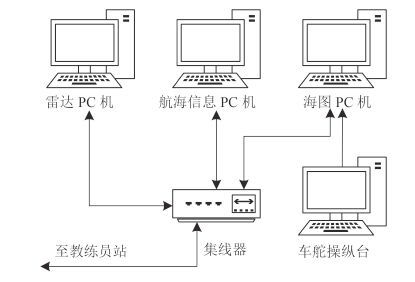图 1 雷达模拟器系统组成 Fig. 1 The composition of radar simulator
1.2 功能设计要求

1） 一般功能：参照 IMO 雷达性能规范进行开发，使其能满足 IMO 关于雷达模拟器的一般性能标准，可用于科研、教学培训等

2） 新增功能：新型雷达模拟器要有全新界面交互系统，要求界面美观、布局合理、操作简单方便。同时实现对新一代雷达新增功能的模拟，使其多功能化。

2 关键模块

2.1 岸线回波和杂波图像生成模块

2.2 偏心显示、量程和显示模式切换模块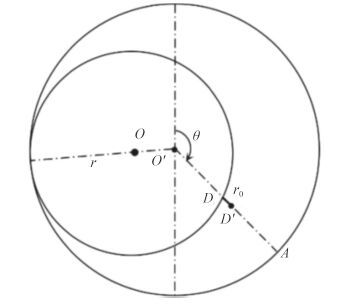图 2 偏心显示时的刻度线端点计算 Fig. 2 The calculation of the endpoints of graduation when off-center display

O'A 的参数方程为：

 $x = \left( {{x_2}-{x_1}} \right)t + {x_1}\text{，}$ (1)
 $y = \left( {{y_2}-{y_1}} \right)t + {y_1}\text{，}$ (2)

O 的参数方程为：

 ${x^2} + {y^2} = {r^2}\text{，}$ (3)

D 位于圆O 上，其坐标满足圆O 的方程，则有

 ${\left[ {\left( {{x_2}-{x_1}} \right)t + {x_1}} \right]^2} + {\left[ {\left( {{y_2}-{y_1}} \right)t + {y_1}} \right]^2} = {r^2}\text{，}$ (4)

 $\begin{array}{l} {r^2}{\rm{ = }}{t^2}\left[ {{{\left( {{x_2}-{x_1}} \right)}^2} + {{\left( {{y_2}-{y_1}} \right)}^2}} \right] + \\ \;\;\;\;\;\;\left[ {2{x_1}\left( {{x_2}-{x_1}} \right) + 2{y_1}\left( {{y_2}-{y_1}} \right)} \right]t + \left( {{x_1} + {y_1}} \right)\text{。} \end{array}$ (5)

 $x{\rm{'}} = O{\rm{'}}D{\rm{'}}\sin \theta + {x_1}\text{，}$ (6)
 $y' =-O'D'\cos \theta + {y_1}\text{。}$ (7)

2.3 雷达刻度信息系统模块

1） 根据 IMO 对船用雷达标准的要求，雷达不同量程对应的 FRM 个数有所不同，当量程变换时每个 FRM 距离也会相应变化。本文依据量程确定 FRM 的个数，确定各距标圈显示圆的半径，进而绘制各固定距标圈。

2） VRM、EBL 和 PI 的生成与 FRM 的类似，但与之不同的是：VRM 可以通过光标控制其半径和中心位置，EBL 可以通过光标控制其端点位置以及方位，PI 可以通过光标实现其旋转和移动。本文通过图像坐标平移实现 VRM，EBL 和 PI 的移动，通过图像坐标旋转实现 EBL 和 PI 的转动，通过图像坐标缩放来实现 VRM 半径的改变。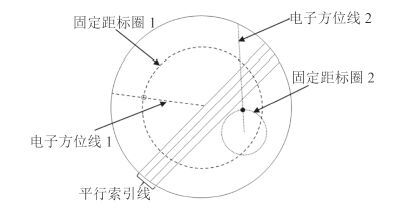图 3 雷达刻度信息系统 Fig. 3 The design of radar information system
2.4 目标跟踪与 AIS 目标报告模块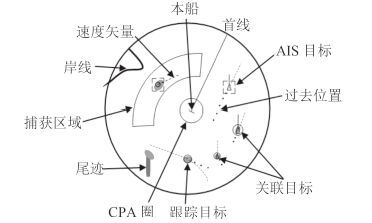图 4 雷达目标跟踪与 AIS 目标报告 Fig. 4 Target tracking and AIS target reporting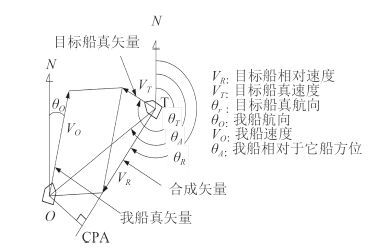图 5 CPA 和 TCPA 的计算 Fig. 5 The calculation of CPA and TCPA
 ${R_T} = OT = \sqrt {{{\left( {{X_O}-{X_T}} \right)}^2} + {{\left( {{Y_O}-{Y_T}} \right)}^2}} \text{；}$ (8)

 ${\theta _A} = {\tan ^{-1}}\left( {\left( {{Y_T}-{Y_O}} \right)/\left( {{X_T}-{X_O}} \right)} \right) + \pi \text{，}$ (9)

 ${CPA} = \left| {{R_T}\sin \left( {{\theta _R}-{\theta _A}} \right)} \right|\text{，}$ (10)
 ${TCPA} = \left| {{R_T}\cos \left( {{\theta _R}-{\theta _A}} \right)} \right|/\left| {{V_R}} \right|\text{。}$ (11)
2.5 新增功能模块 2.5.1 控制面板的开发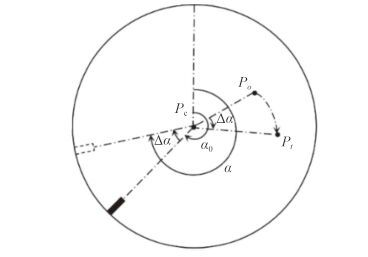图 6 旋钮的实现 Fig. 6 The realization of knob

1） 定义 Point（点）变量 Po，Pt，Pc 以及旋钮初始旋转角度 α0

2） 当鼠标进入旋钮并开始拖动时获取鼠标在旋钮上初始坐标 Po

3） 在鼠标拖动后获取鼠标在旋钮上的坐标 Pt。分别求取 PoPt 与旋钮中心 Pc 的向量$\overrightarrow{{{P}_{o}}{{P}_{c}}}$和$\overrightarrow{{{P}_{t}}{{P}_{c}}}$，并求出两向量的夹角：

 $\Delta {\rm{\alpha }} = {\cos ^{-1}}\left( {\overrightarrow {{P_o}{P_c}} \cdot \overrightarrow {{P_t}{P_c}} /\left( {\left| {\overrightarrow {{P_o}{P_c}} } \right| \cdot \left| {\overrightarrow {{P_t}{P_c}} } \right|} \right)} \right)\text{。}$ (12)

4） 重复进行第 3 步，从而实现旋钮的旋转。

2.5.2 深度指示器、风向/风速指示器、航向舵角指示 器、温度指示器的开发

2.5.3 系统语言切换

2.5.4 统一共同基准点的设置

2.5.5 捕获区域的模拟

AZ（Acquisition/Activation Zone，捕获区域）一直是船舶导航雷达的强制性能标准，目前各雷达模拟器也均实现了此功能。但目前捕获区域的设置均较为复杂，模拟效果不好。本文使用了一种新的捕获区域设置方法：首先显示捕获区域，移动光标到捕获区域边线上，光标形状由十字变为箭头，左击捕获区域边线，其上出现 8 个白色基准点，拖动基准点即可调整捕获区域位置、形状。当捕获区域到达指定位置或理想形状时，单击捕获区域外任意位置，即可完成捕获区域设置。本文通过碰撞检测判断目标是否进入或离开捕获区域，以实现捕获区域自动捕获、激活等功能。

3 仿真实现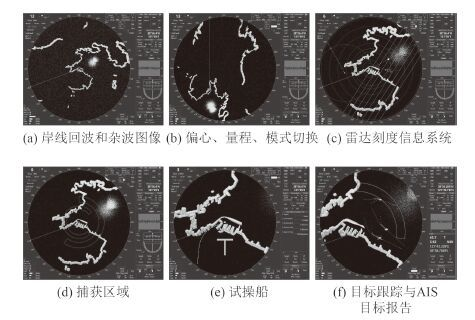图 7 雷达模拟器主功能界面 Fig. 7 The main interface of radar simulator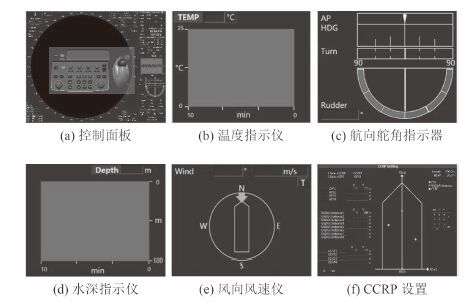图 8 部分新增功能 Fig. 8 Some new functions
4 结 语

  杜芳磊. 训练模拟器雷达模拟技术的研究[D]. 哈尔滨:哈尔滨工程大学, 2012. http://cn.bing.com/academic/profile?id=83a1098197c402f722587584c0a15f84&encoded=0&v=paper_preview&mkt=zh-cn  刘荣兰. 综合船桥系统中基于电子海图的导航信息融合技术研究[D]. 武汉:武汉理工大学, 2013. http://cn.bing.com/academic/profile?id=9b190343627d48b174054201ca2900dc&encoded=0&v=paper_preview&mkt=zh-cn  IMO. Resolution MSC. 192(79):Revised Recommendation on Performance Standards for Radar Equipment[R]. London:IMO, 2004.  张杨, 曾浩, 察豪, 等. 国外航海雷达现状及发展趋势[J]. 电讯技术, 2013 (12):1649–1653.  倪德山. 航海雷达模拟器训练[M]. 大连: 大连海事大学出版社, 2011.  MMACDONALD M. Pro WPF in C# 2010:Windows Presentation Foundation in. NET 4.0[M]. Berkeley: Apress, 2010.  中华人民共和国海事局. 1978年海员培训、发证和值班标准国际公约马尼拉修正案[M]. 大连: 大连海事大学出版社, 2010.  郭禹. 航海学[M]. 大连: 大连海事大学出版社, 2005.  VINCENTY T. Direct and inverse solutions of geodesics on the ellipsoid with application of nested equations[J]. Survey Review, 1975, 23 (176):88–93. DOI: 10.1179/sre.1975.23.176.88  PRASAD D K, LEUNG M K H, CHAI Q, et al. A novel frame-work for making dominant point detection methods non-parame-tric[J]. Image and Vision Computing, 2012, 30 (11):843–859. DOI: 10.1016/j.imavis.2012.06.010  尹勇, 刘秀文, 李志华. 采用真雷达显示器的航海雷达模拟器的关键技术[J]. 系统仿真学报, 2007, 19 (5):1014–1017.  张润泽. 船舶导航雷达(第三册)[M]. 北京: 人民交通出版社, 1990.  金一丞, 尹勇. 航海模拟器[M]. 北京: 科学出版社, 2013.  吴兆麟, 赵月林. 船舶避碰与值班(第三版)[M]. 大连: 大连海事大学出版社, 2008.  陈大军, 任鸿翔, 肖方兵. 语音识别技术在船舶甚高频仿真设备中的应用[J]. 大连海事大学学报, 2014, 40 (2):73–76.